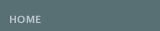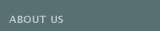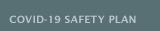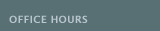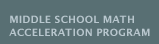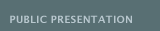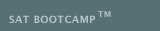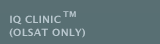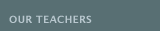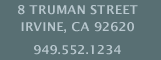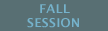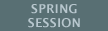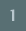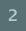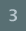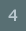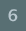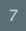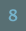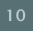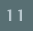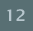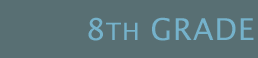The CRITICAL WRITING FOR THE 8th GRADE is a small group class that provides instruction aligned with the California's Common Core State Standards in the following areas: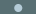Active Reading (Reading Comprehension): Students will read and understand 4-6 novels, cite textual evidence that most strongly supports analysis and inferences, determine a central idea including its relationship to the characters, analyze the relevance of the setting (e.g., time, place, customs) to the mood, tone, and meaning of the text, analyze recurring themes (e.g., good versus evil) across traditional and contemporary works, provide an objective summary, and analyze significant literary devices (e.g., metaphor, symbolism, dialect, irony) that define a writer�s style and use those elements to interpret the work.Fundamentals of Effective Writing: Students will be writing clear, coherent, and focused essays that introduce a strong thesis statement that clearly previews what is to follow, organize concepts and information with logical reasoning and relevant evidence, use phrases and clauses to create cohesion and clarify the relationships among reasons, use precise language to establish and maintain a formal style and provide a conclusion that follows from and supports the argument presented. Students will be practicing the three writing genres (expository, persuasive arguments, and descriptive narratives) to include at least 500�1000 words in each composition.Vocabulary Development: Students will integrate knowledge of individual words to enhance their writing, analyze idioms, analogies, metaphors, and similes to infer the literal and figurative meanings of phrases, and use word meanings within the appropriate context and show ability to verify those word meanings by definition, example, restatement, comparison, or contrast.Grammar: For the grammar module of the class, students will learn to use correct and varied sentence types and sentence opening to present a lively and effective personal style, identify and use parallelism, form and use verbs in the indicative, imperative, interrogative, conditional, and subjunctive mood, present items in a series and items juxtaposed for emphasis, and use subordination, coordination, apposition, and other devices to indicate clearly the relationship between ideas. Some book purchases will be necessary. Students can expect homework and weekly quizzes. Grades will be given.
 E08A CRITICAL WRITING (8th Gr) August 15�January 20 (23 weeks)* *November 21�25 (Thanksgiving Recess) *December 19�January 2 (Winter Recess)
 Wednesdays 4:00�6:00pm LEE
 MATH 8 (MSMAP) is designed for the end of course (EOC) test for MATH 1 placement the following year. This course introduces the language and methods of algebra. Instruction will focus on 4 critical areas:Expressions and Equations: Solve real-world and mathematical problems of two linear equations in two variables algebraically, and estimate solutions by graphing the equations.Functions: Interpret the equation y=mx+b as defining a linear function. Construct a function to model a linear relationship between two quantities.Geometry: Apply the Pythagorean Theorem to determine unknown side lengths in right triangles in real-world and mathematical problems in two and three dimensions. Apply the formulas for the volumes of cones, cylinders, and spheres and use them to solve real-world and mathematical problems.Statistics and Probability: Construct and interpret scatter plots for bivariate measurement data to investigate patterns of association between two quantities.
 Students will be required to attend twice a week (one lesson to emphasize problem solving and word problems and the other to reinforce accuracy). Students are expected to complete additional exercise problem sets for homework. Students can expect homework, quizzes, a mid-term and a final. Grades will be given.
 All seventh graders must first pass the end of year test in order to take Math 1 Acceleration Test in the Spring.
 M01A Math 8 (Math 1 Placement-MSMAP)
 August 15�January 20 (22 weeks)* *November 21�25 (Thanksgiving Recess) *December 19�January 2 (Winter Recess)
 Thursdays 4:00-6:00pm KELLY
 December 7�January 18 (5 weeks)* *September 5 (Labor Day Observed) *October 10 (Columbus Day Observed) *November 21�25 (Thanksgiving Recess) *December 19�January 2 (Winter Recess) *January 16 (Martin Luther King Day Observed)
 Mondays or Wednesdays 4:00-6:00pm KELLY
 In ENHANCED MATH 1 at the BARUN ACADEMIC CENTER students will learn to reason abstractly and quantitatively. Instruction will highlight 4 critical areas:Algebra: Represent and solve equations and inequalities graphically. Explain why the x-coordinates of the points where the graphs of the equations y=f(x) and y=g(x) intersect are the solutions of the equation f(x)=g(x). Include cases where f(x) and/or g(x) are linear, polynomial, rational, absolute value, exponential, and logarithmic functions.Functions: Calculate and interpret the average rate of change of a function (presented symbolically or as a table) over a specified interval. Estimate the rate of change from a graph.Geometry: Develop definitions of rotations, reflections, and translations in terms of angles, circles, perpendicular lines, parallel lines, and line segments. Prove the slope criteria for parallel and perpendicular lines and use them to solve geometric problems.Statistics and Probability: Summarize categorical data for two categories in two-way frequency tables. Fit a function to the data. This group class is for the student enrolled in Math 1 (Integrated). Students can expect homework, quizzes, a mid-term and a final. Grades will be given.
 M02A ENHANCED MATH 1 August 15�January 20 (23 weeks)* *September 5 (Labor Day Observed) *October 10 (Columbus Day Observed) *November 21�25 (Thanksgiving Recess) *December 19�January 2 (Winter Recess) *January 16 (Martin Luther King Day Observed)
 Mondays 4:00�6:00pm KELLY
 In ENHANCED MATH 2 at the BARUN ACADEMIC CENTER, students will learn to reason abstractly and quantitatively, attend to precision, and model with mathematics. Instruction will highlight 4 critical areas:Algebra: Solve quadratic equations by inspection (e.g., for x2=49), taking square roots, completing the square, the quadratic formula, and factoring, as appropriate to the initial form of the equation. Recognize when the quadratic formula gives complex solutions and write them as a ± bi for real numbers a and b.Functions: Prove the Pythagorean identity sin2(θ) + cos2(θ) = 1 and use it to find sin(θ), or tan(θ) given sin(θ), cos(θ), or tan(θ) and the quadrant of the angle.Geometry: Verify experimentally that in a triangle, angles opposite longer sides are larger, sides opposite larger angles are longer, and the sum of any two sides lengths is greater than the remaining side length; apply these relationships to solve real-world and mathematical problems.Statistics and Probability: Apply the Addition Rule, P(A or B) = P(A) + P(B) - P(A and B), and interpret the answer in terms of the model. This group class is for the student enrolled in Enhanced Math 2. Students can expect homework, quizzes, a mid-term and a final. Grades will be given.
 M03A ENHANCED MATH 2
 August 15�January 20 (23 weeks)* *September 5 (Labor Day Observed) *October 10 (Columbus Day Observed) *November 21�25 (Thanksgiving Recess) *December 19�January 2 (Winter Recess) *January 16 (Martin Luther King Day Observed)
 Mondays 4:00�6:00pm SINGLETON